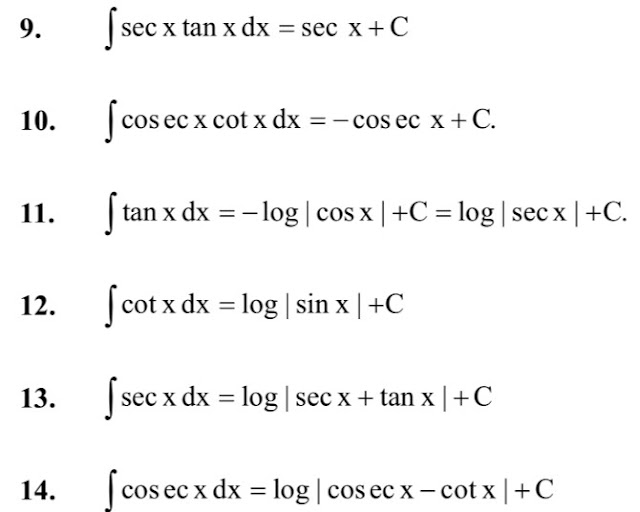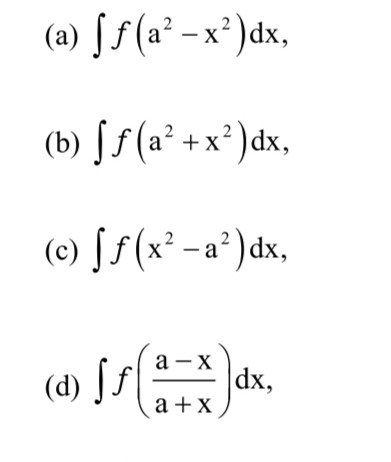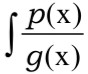12 class Maths Notes Chapter 7 Integrals free PDF| Quick revision Integrals Notes class 12 maths

CBSE Revision Notes for CBSE Class 12 Mathematics Integrals Integration as inverse process of differentiation.Integration of a variety of functions by substitution, by partial fractions and by parts, Evaluation of simple integrals of the following types and problems based on them. Definite integrals as a limit of a sum, Fundamental Theorem of Calculus (without proof).Basic properties of definite integrals and evaluation of definite integrals.

Class 12 Maths Chapter-7 Integrals Quick Revision Notes Free Pdf

🔷 Chapter - 7 🔷
👉  Integrals 👈

Integration is the inverse process of differentiation. That is, the process of finding a function, whose differential coefficient is known, is called integration.

If the differential coefficient of F(x) is f (x),

or integral of f (x) is F(x), written as ∫ f (x)dx = F(x),

Here ∫ dx is the notation of integration f (x) is the integrand, x is the variable of integration and dx denotes the integration with respect to x.

✳️ INDEFINITE INTEGRAL

This shows that F(x) and F(x) +C are both integrals of the same function f(x). Thus, for different values of C, we obtain different integrals of f(x). This implies that the integral of f(x) is not definite. By virtue of this property F(x) is called the indefinite integral of f(x).

✳️  Properties of Indefinite Integration

✳️ Standard Formulae of Integration

The following results a direct consequence of the definition of an integral.✳️ 2 . METHODS OF INTEGRATION

✳️ 2 . 1 Method of Substitution

By suitable substitution, the variable x in ∫ f(x)dx is changed into another variable t so that the integrand f (x) is changed into F(t) which is some standard integral or algebraic sum of standard integrals.

There is no general rule for finding a proper substitution and the best guide in this matter is experience.

However, the following suggestions will prove useful.

✳️ 2.1.1 Some Special Integrals

✳️ 2.1.2 Integrals of the Form✳️ Working Rule

✳️ 2.1.3 Integrals of the Form

✳️ Working Role

(i) Make the coefficient of x² unity by taking the coefficient of x² outside the quadratic.

(ii) Complete the square in the terms involving x, i.e. write ax² + bx + c in the form

(iii) The integrand is converted to one of the nine special integrals.

(iv) Integrate the function.

✳️ 2.1.4  Integrals of the Form

✳️ Integral Working Rule

✳️ 2.1.5 Integrals of the Form

where k, a0, a1, ... an-1, are constants to be determined by differentiating the above relation and equating the coefficients of various powers of x on both sides.

✳️ 2.1.6 Integrals of the Form

where k is a constant positive, negative or zero.

Working Rule

(i) Divide the numerator and denominator by x².

(iii) Evaluate the resulting integral in z

(iv) Express the result in terms of x.

✳️ 2.1.7 Integrals of the Form

✳️ METHOD OF PARTIAL FRACTIONS FOR RATIONAL FUNCTIONS

Integrals of the typecan be integrated by resolving the integrand into partial fractions. We proceed as follows:
Check degree of p (x) and g (x).

If degree of p (x) ≥ degree of g (x), then divide p (x) by g (x) till its degree is less, i.e. put in the

where A₁, A₂, ... Aₙ are constants to be determined by comparing the coefficients of various powers of x on both sides after taking L.C.M.

CASE 2: When the denominator contains repeated as well as non-repeated linear factor. That is
where A₁, A₂, ... Aₙ are constants to determined by comparing the coefficients of various powers of x on both sides after taking L.C.M.

Note : Corresponding to repeated linear factor (x - a)r in the denominator, a sum of r partial

CASE 3: When the denominator contains a non repeated quadratic factor which cannot be factorised further:

g (x) = (ax² + bx + c) (x - a₃) (x - a₄)... (x - aₙ).

In such a case express f (x) and g (x) as:

where A₁, A₂, ... Aₙ are constants to be determined by comparing the coefficients of various powers of x on both sides after taking L.C.M.

CASE 4: When the denominator contains a repeated quadratic factor which cannot be factorised further: That is

g (x) = (ax² + bx + c)² (x - a₅) (x - a₆) .. (x aₙ)
In such a case write f(x) and g (x) as

where A₁, A₂, .. Aₙ are constants to be determined by comparing the coefficients of various powers of x on both sides after taking L.C.M.

CASE 5: If the integrand contains only even powers of x

(i) Put x² = z in the integrand.
(ii) Resolve the resulting rational expression in z into partial fractions
(iii) Put z = x² again in the partial fractions and then integrate both sides.

✳️ METHOD OF INTEGRATION BY PARTS

The process of integration of the product of two functions is known as integration by parts.

For example, if u and v are two functions of x,
In words, integral of the product of two functions = first function x integral of the second - integral of (differential of first x integral of the second function).

Working Hints

(i) Choose the first and second function in such a way that derivative of the first function and the integral of the second function can be easily found.

(ii) In case of integrals of the form ∫ f(x).xⁿ dx, take xⁿ as the first function and f (x) as the second function.

(iii) In case of integrals of the form ∫(logx)ⁿ. 1 dx, take 1 as the second function and (log x)ⁿ as the first function.

(iv) Rule of integration by parts may be used repeatedly, if required.

(v) If the two functions are of different type, we can choose the first function as the one whose initial comes first in the word "ILATE", where

I - Inverse Trigonometric function
L- Logarithmic function
A- Algebraic function
T- Trigonometric function
E- Exponential function.

(vi) In case, both the functions are trigonometric, take that function as second function whose integral is simple. If both the functions are algebraic, take that function as first function whose derivative is simpler.

(vii) If the integral consists of an inverse trigonometric function of an algebraic expression in x, first simplify the integrand by a suitable trigonometric substitution and then integrate the new integrand.

4.1 Integrals of the Form

∫eˣ [f(x)+ f´(x)]dx

Working Rule

(i) Split the integral into two integrals.
(ii) Integrate only the first integral by parts, i.e.

∫eˣ [f(x)+ f´(x)] x =∫eˣ f(x) x+ ∫eˣf´(x) dx

=[ F(x).eˣ - ∫f'(x).eˣ x] +∫eˣ f'(x) dx

=eˣf(x)+C

4.2 Integrals of the Form:

Where the initial integrand reappears after integrating by parts.

Working Rule

(i) Apply the method of integration by parts twice.

(ii) On integrating by parts second time, we will obtain the given integrand again, put it equal to I.

(iii) Transpose and collect terms involving I on one side and evaluate I.

✳️ INTEGRAL OF THE FORM (TRIGONOMETRIC FORMATS)

Working Rule

(i) Divide the numerator and denominator by cos²x.
(ii) In the denominator, replace sec²x, if any, by 1 + tan²x.
(iii) Put tan x = z >⇒ sec²x dx = dz.
(iv) Integrate the resulting rational algebraic function of z.
(v) In the answer, put z = tan x.

5.3 Integrals of the Form

5.4 Integrals of the Form

5.5 Integrals of the Form

Working Rule

(i) If the power of sin x is an odd positive integer, put cos x = t.
(ii) If the power of cos x is an odd positive integer, put sin x = t.
(iii) If the power of sin x and cos x are both odd positive integers, put sin x = t or cos x = t.

(iv) If the power of sin x and cos x are both even positive integers, use De' Moivre's theorem as follows:

Now expand each of the factors on the R.H.S. using Binomial theorm. Then group the terms equidistant from the beginning and the end. Thus express all such pairs as the sines or cosines of multiple angles. Further integrate term by term.

(v) If the sum of powers of sin x and cos x is an even negative integer, put tan x = z.

SLOVED EXAMPLES